# Schedules of Reinforcement and Choice Simple Schedules Ratio

• Slides: 29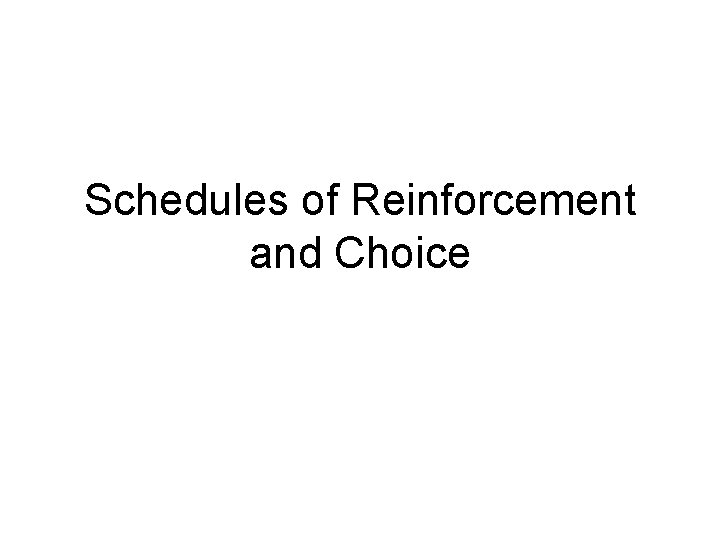Schedules of Reinforcement and ChoiceSimple Schedules • • Ratio Interval Fixed Variable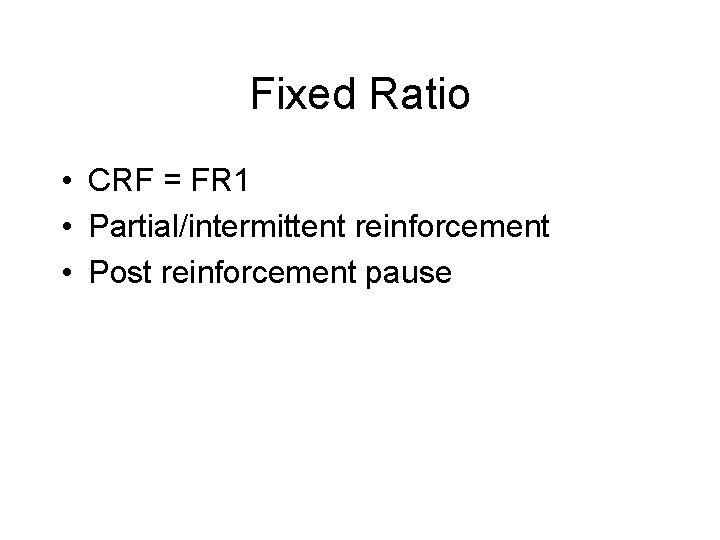Fixed Ratio • CRF = FR 1 • Partial/intermittent reinforcement • Post reinforcement pauseCauses of FR PRP • Fatigue hypothesis • Satiation hypothesis • Remaining-responses hypothesis – Reinforcer is a discriminative stimulus signaling absence of next reinforcer any time soon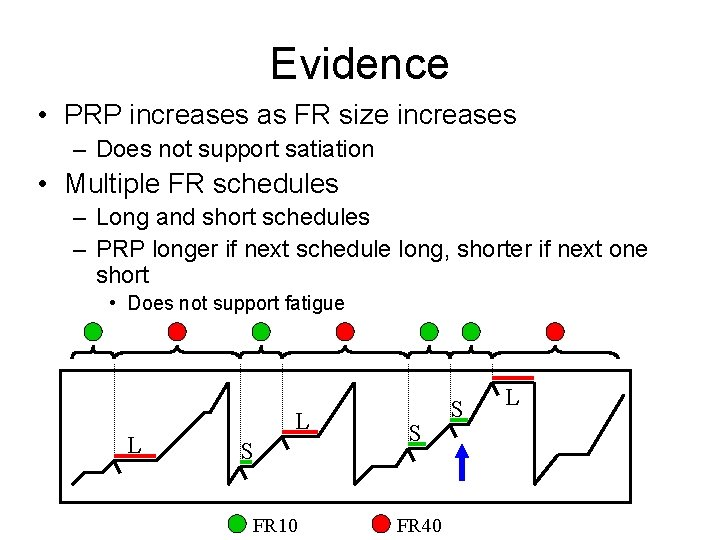Evidence • PRP increases as FR size increases – Does not support satiation • Multiple FR schedules – Long and short schedules – PRP longer if next schedule long, shorter if next one short • Does not support fatigue L L S FR 10 S FR 40 S L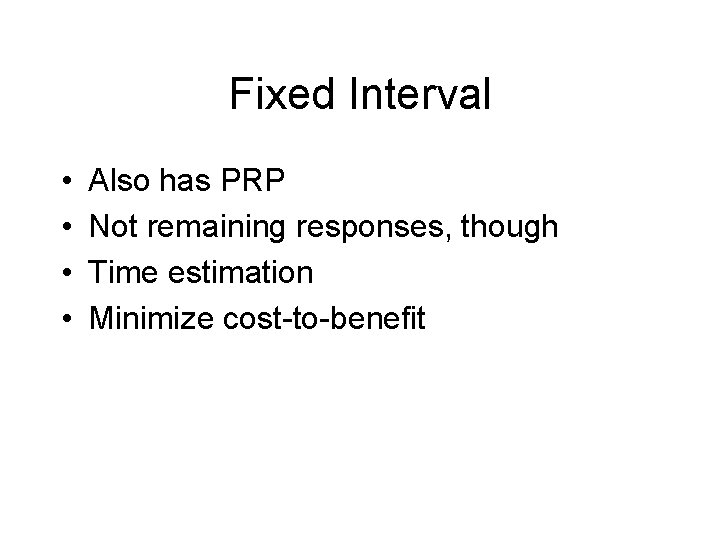Fixed Interval • • Also has PRP Not remaining responses, though Time estimation Minimize cost-to-benefit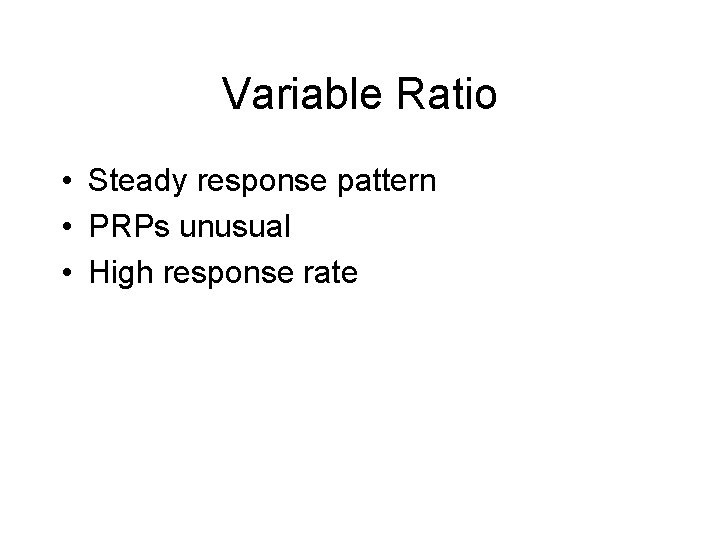Variable Ratio • Steady response pattern • PRPs unusual • High response rate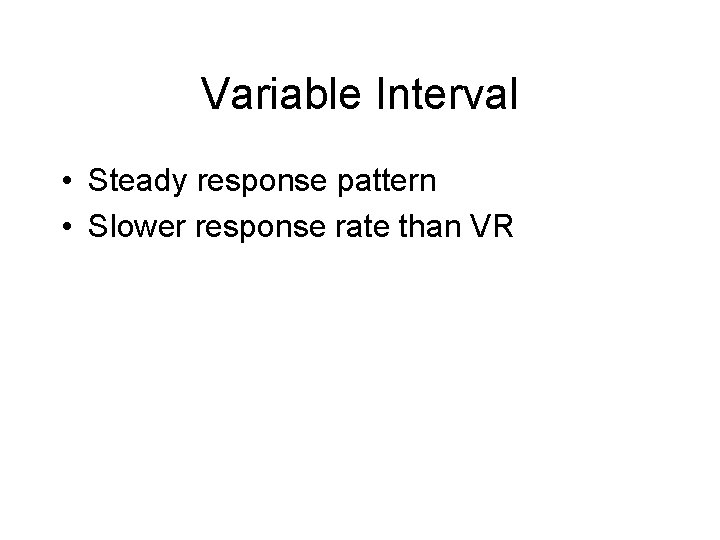Variable Interval • Steady response pattern • Slower response rate than VR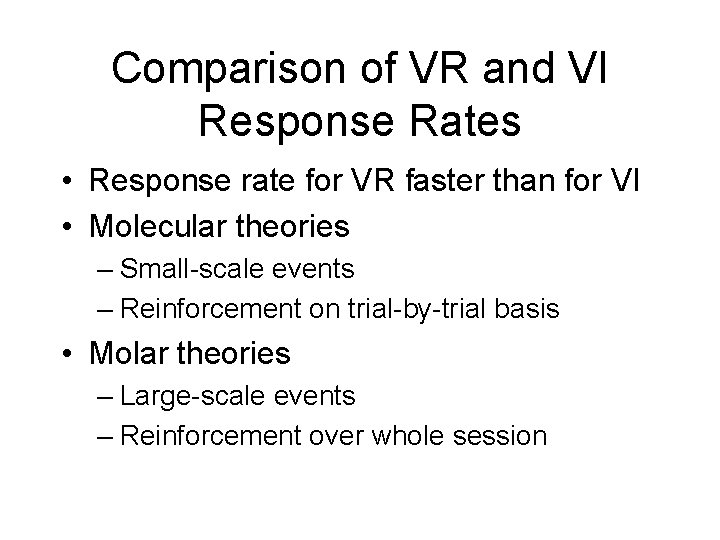Comparison of VR and VI Response Rates • Response rate for VR faster than for VI • Molecular theories – Small-scale events – Reinforcement on trial-by-trial basis • Molar theories – Large-scale events – Reinforcement over whole session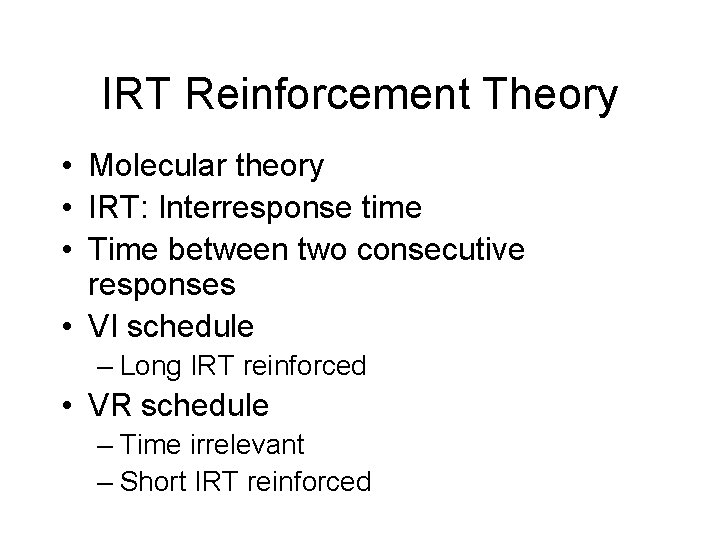IRT Reinforcement Theory • Molecular theory • IRT: Interresponse time • Time between two consecutive responses • VI schedule – Long IRT reinforced • VR schedule – Time irrelevant – Short IRT reinforced• Random number generator (mean=5) • 30 reinforcer deliveries Time/number for reinforcement 895323566436145668… Time b/t responses 1 3 10 4 1 1 1 3 8 8 7 9 9 7 1 1 5 6 1 9 8 5 1 4 1 9 6 3 10 5 … i i i i r r r Ratio number Interval 1 2 3 4 5 6 seconds 7 8 9 10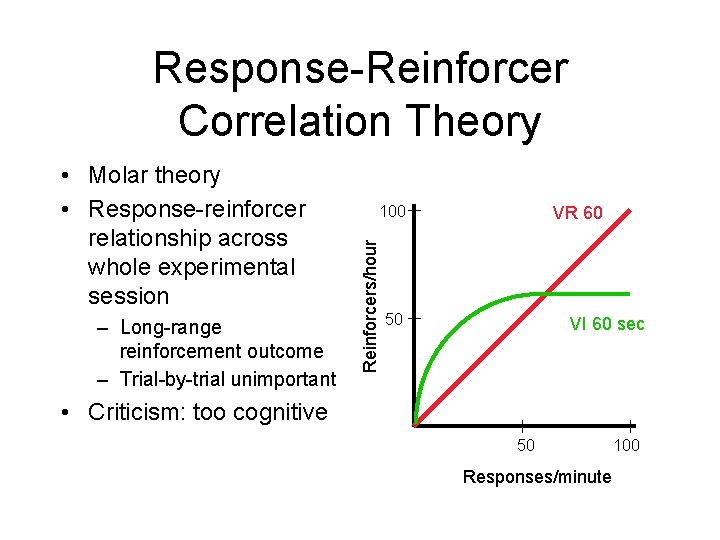Response-Reinforcer Correlation Theory – Long-range reinforcement outcome – Trial-by-trial unimportant 100 Reinforcers/hour • Molar theory • Response-reinforcer relationship across whole experimental session VR 60 50 VI 60 sec • Criticism: too cognitive 50 Responses/minute 100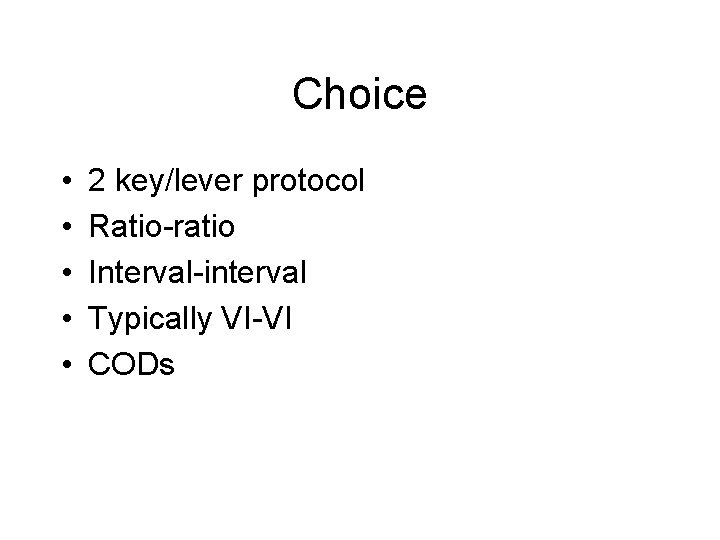Choice • • • 2 key/lever protocol Ratio-ratio Interval-interval Typically VI-VI CODsMatching Law B 1 + B 2 = R 1 + R 2 or • B = behaviour (responses) • R = reinforcement B 1 B 2 = R 1 R 2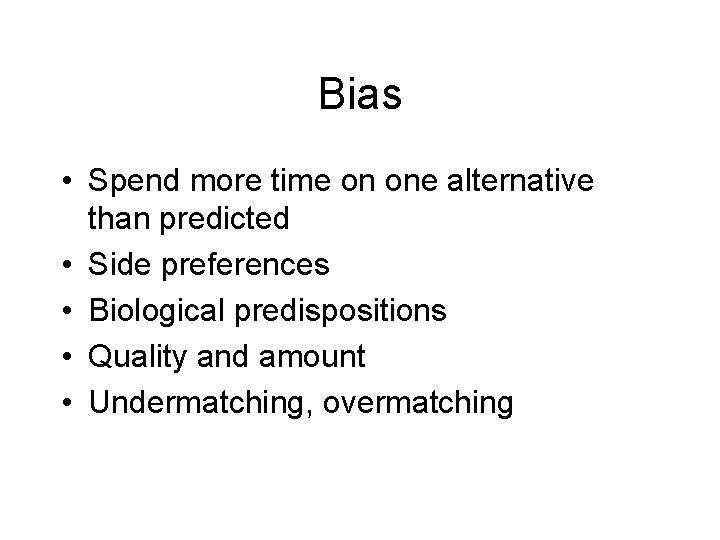Bias • Spend more time on one alternative than predicted • Side preferences • Biological predispositions • Quality and amount • Undermatching, overmatchingQualities and Amounts • • Q 1: quality of first reinforcer Q 2: quality of second reinforcer A 1: amount of first reinforcer A 2: amount of second reinforcerUndermatching • Most common • Response proportions less extreme than reinforcement proportionsOvermatching • Response proportions are more extreme than reinforcement proportions • Rare • Found when large penalty imposed for switching – e. g. , barrier between keysUndermatching/Overmatching Undermatching 1 B 1+B 2 1 0. 5 0 0. 5 R 1 R 1+R 2 1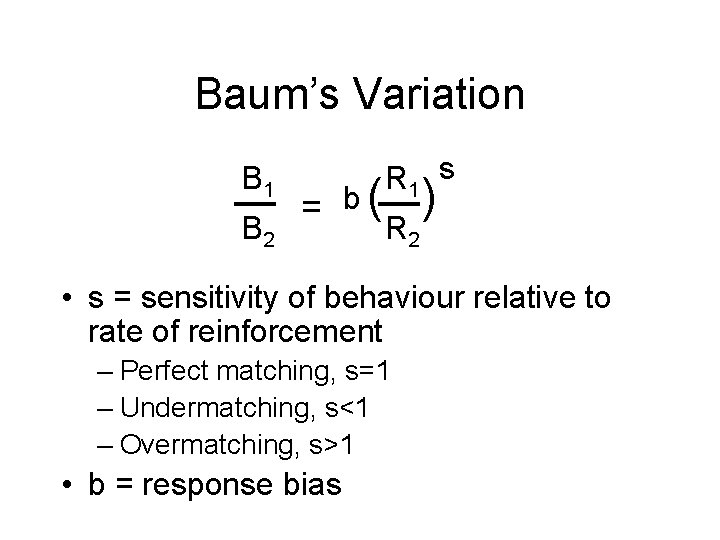Baum’s Variation B 1 B 2 = b( R 1 s R 2 ) • s = sensitivity of behaviour relative to rate of reinforcement – Perfect matching, s=1 – Undermatching, s<1 – Overmatching, s>1 • b = response biasMatching as a Theory of Choice • Animals match because they are evolved to do so. • Nice, simple approach, but ultimately wrong. • Consider a VR-VR schedule – Exclusively choose one alternative • Whichever is lower – Matching law can’t explain this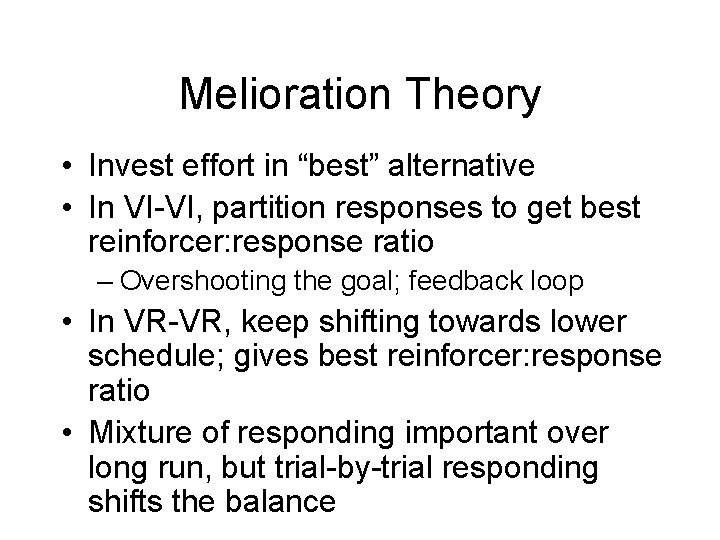Melioration Theory • Invest effort in “best” alternative • In VI-VI, partition responses to get best reinforcer: response ratio – Overshooting the goal; feedback loop • In VR-VR, keep shifting towards lower schedule; gives best reinforcer: response ratio • Mixture of responding important over long run, but trial-by-trial responding shifts the balanceOptimization Theory • Optimize reinforcement over long-term • Minimum work for maximum gain • Respond to both choices to maximize reinforcement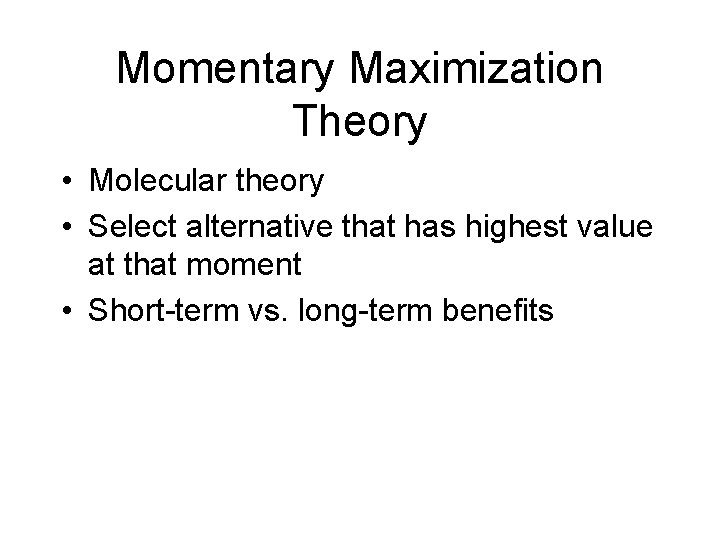Momentary Maximization Theory • Molecular theory • Select alternative that has highest value at that moment • Short-term vs. long-term benefitsDelay-reduction Theory • Immediate or delayed reinforcement • Basic principles of matching law, and. . . • Choice directed towards whichever alternative gives greatest reduction in delay to next reinforcer • Molar (matching response: reinforcement) and molecular (control by shorter delay) features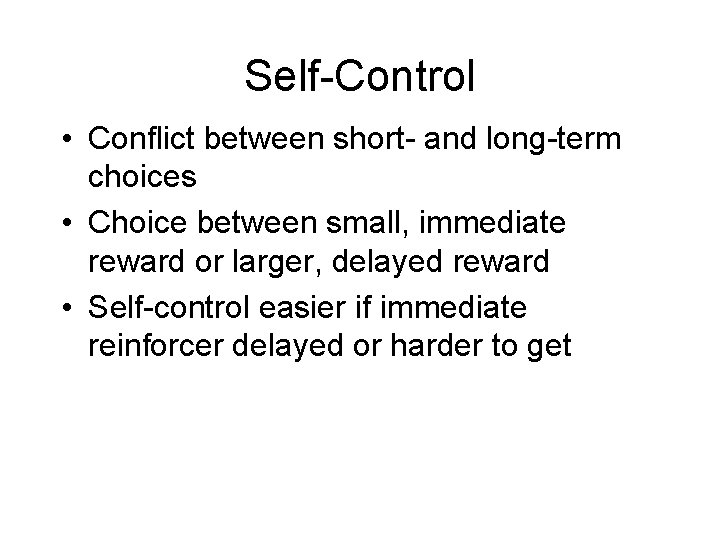Self-Control • Conflict between short- and long-term choices • Choice between small, immediate reward or larger, delayed reward • Self-control easier if immediate reinforcer delayed or harder to get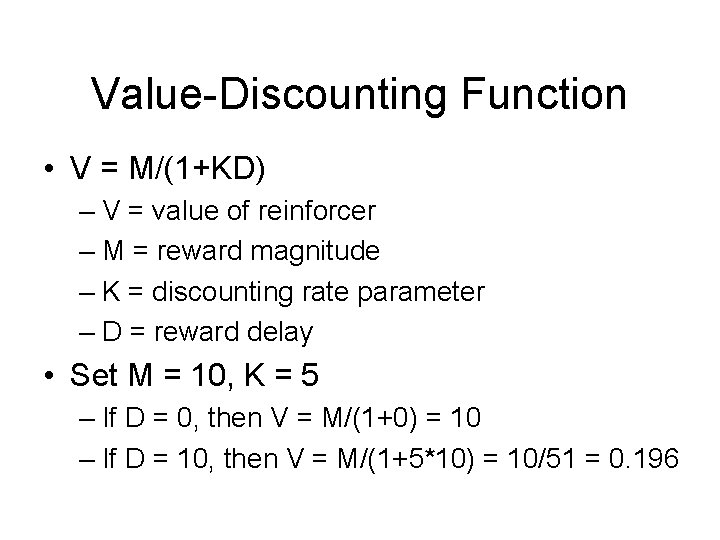Value-Discounting Function • V = M/(1+KD) – V = value of reinforcer – M = reward magnitude – K = discounting rate parameter – D = reward delay • Set M = 10, K = 5 – If D = 0, then V = M/(1+0) = 10 – If D = 10, then V = M/(1+5*10) = 10/51 = 0. 196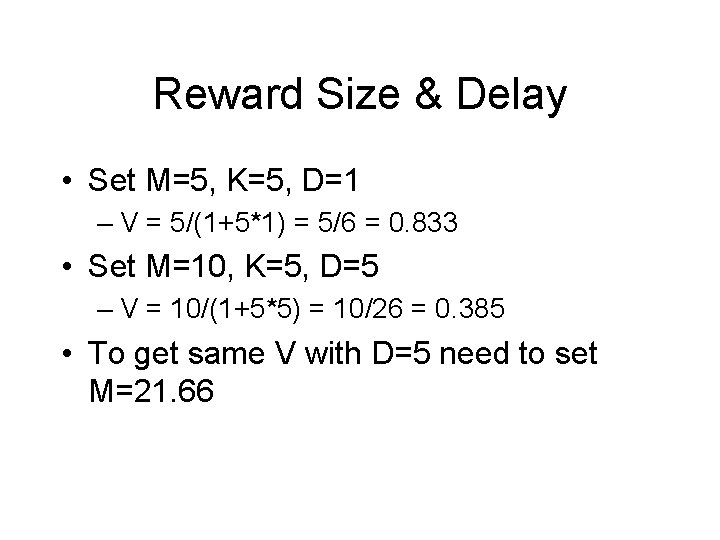Reward Size & Delay • Set M=5, K=5, D=1 – V = 5/(1+5*1) = 5/6 = 0. 833 • Set M=10, K=5, D=5 – V = 10/(1+5*5) = 10/26 = 0. 385 • To get same V with D=5 need to set M=21. 66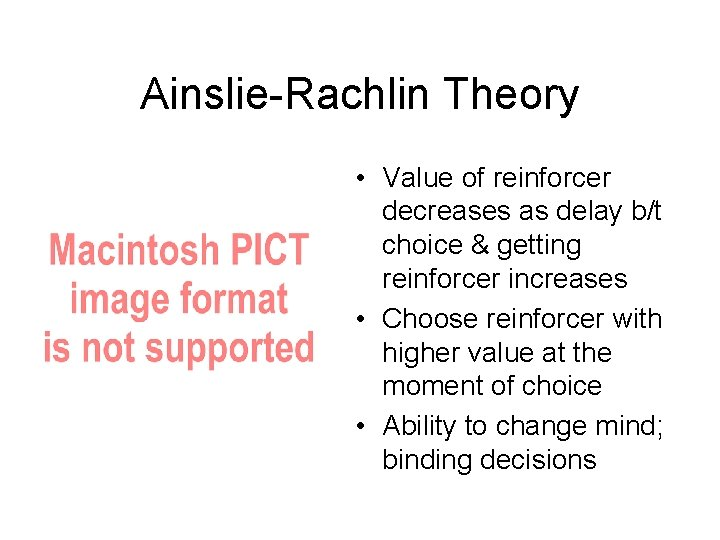Ainslie-Rachlin Theory • Value of reinforcer decreases as delay b/t choice & getting reinforcer increases • Choose reinforcer with higher value at the moment of choice • Ability to change mind; binding decisions